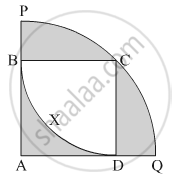Advertisement Remove all ads

# In the Given Figure, Square Abcd is Inscribed in the Sector a - Pcq. the Radius of Sector C - Bxd is 20 Cm. Complete the Following Activity to Find the Area of Shaded Region - Geometry

Advertisement Remove all ads
Advertisement Remove all ads
Advertisement Remove all ads
SumIn the given figure, square ABCD is inscribed in the sector A - PCQ. The radius of sector C - BXD is 20 cm. Complete the following activity to find the area of shaded region

Advertisement Remove all ads

#### Solution

Side of square ABCD = radius of sector C-BXD = 20 cm

Area of square = (side)2 = 20= 400 cm

Area of shaded region inside the square
= Area of square ABCD − Area of sector C-BXD
= 400  $- \frac{\theta}{360° } \times \pi r^2$

= 400  $- \frac{90° }{360° } \times \frac{3 . 14}{1} \times \frac{400}{1}$

= 400 - 314

= 86 cm2

Radius of bigger sector = Length of diagonal of square ABCD  = $20\sqrt{2}$ cm

Area of the shaded regions outside the square
= Area of sector A-PCQ − Area of square ABCD
= A(A-PCQ) − A( ABCD )

=  $\frac{\theta}{360° } \times \pi \times r^2$ - 20

= $\frac{90°}{360°} \times 3 . 14 \times \left( 20\sqrt{2} \right)^2$ - (20)

= 628 - 400

= 228 cm

∴ Total surface area of the shaded region = 86 + 228 = 314 cm2

Concept: Surface Area of a Combination of Solids
Is there an error in this question or solution?

#### APPEARS IN

Balbharati Mathematics 2 Geometry 10th Standard SSC Maharashtra State Board
Chapter 7 Mensuration
Practice set 7.4 | Q 11 | Page 162

#### Video TutorialsVIEW ALL 

Advertisement Remove all ads
Share
Notifications

View all notifications

Forgot password?